﻿ 基于CDMA网络的船舶在线式监控系统开发
 舰船科学技术2022, Vol. 44Issue (5): 142-145    DOI: 10.3404/j.issn.1672-7649.2022.05.030PDF

1. 太原科技大学 计算机科学与技术学院，山西 太原 030024;
2. 河南科技职业大学 信息工程学院，河南 周口 466000

Development of ship online monitoring system based on CDMA network
ZHANG Jian-ying1,2, JIANG Xiao-rong2
1. School of Computer Science and Technology, Taiyuan University of Science and Technology, Taiyuan 030024, China;
2. College of Information Engineering, Henan Vocational University of Science and Technology, Zhoukou 466000, China
Abstract: The function of the on-line monitoring system is to collect the position information of the ship, the operation parameter information of electromechanical equipment, the navigation state information of the ship, obtain the real-time working state of the ship through the analysis and processing of the information, and monitor whether there is any abnormality in the operation state of the ship. Therefore, the timeliness of information has a key impact on the performance of ship monitoring system. CDMA is a third generation mobile communication network technology. Combined with CDMA network technology, this paper realizes the accurate positioning of mobile ship terminal, and designs a ship online remote monitoring system combined with CDMA network, which has high practical application value.
Key words: CDMA network     online monitoring     positioning technology     error analysis
0 引　言

1 CDMA网络在船舶终端定位的应用 1.1 CDMA网络通信技术

CDMA码分多址通信是一种利用码序列相关性进行多址通信的技术，其核心内容是利用地址码序列的差异性，得到不同的地址信息，用户从某一个位置发送载波信号时，信号的调制过程不仅要经过数字信号调制，还需要经过地址码的调制。信号接收时，只有特定地址码的CDMA终端才能接收特定的信号，其他终端无法实现信号的解调

CDMA通信具有以下特性：

1）网络数据传输性能广，支持语音信号、电路数据等多类型数据的快速传输。

2）具有复合业务，包括通信、多媒体服务、定位业务等。

3）可实现现有通信系统的平顺切换，具备IS-95通信系统的基础功能。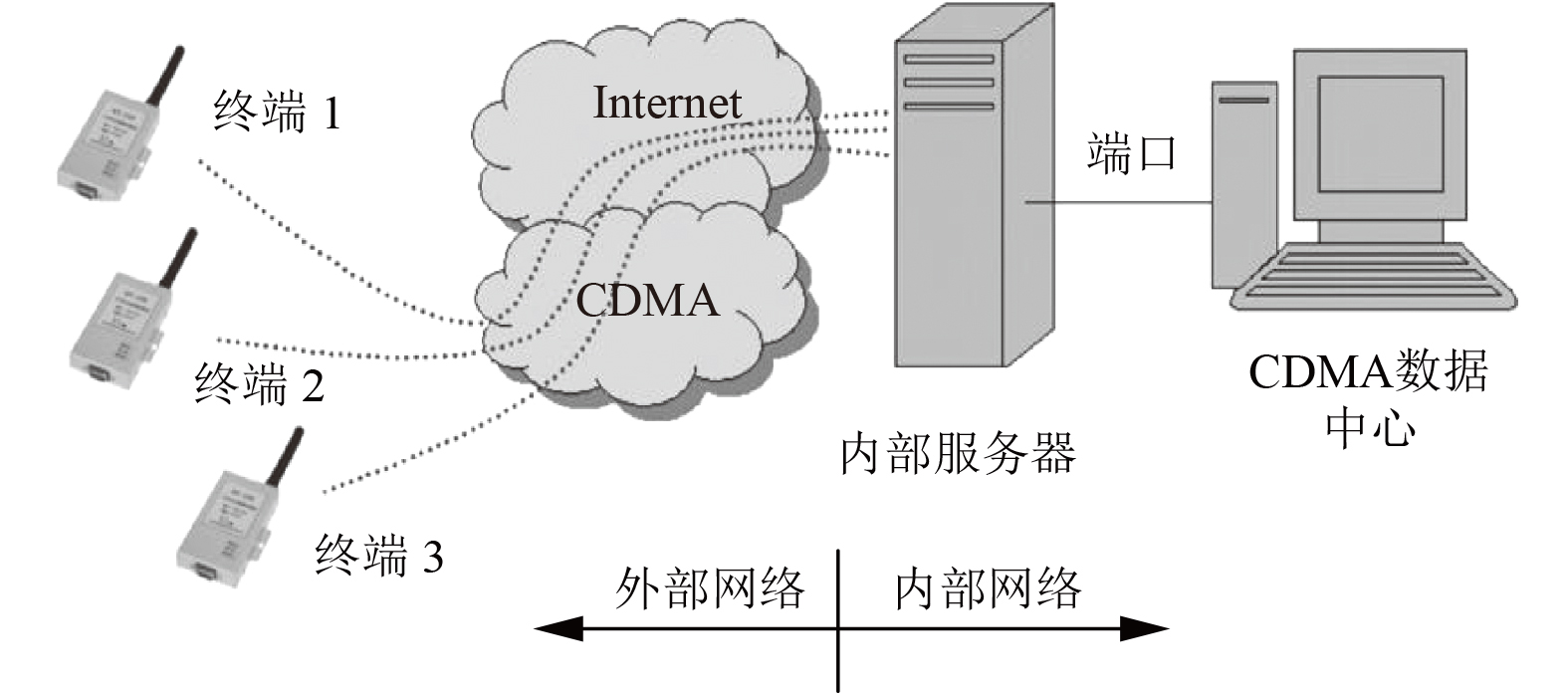图 1 CDMA通信网络的原理图 Fig. 1 Schematic diagram of CDMA communication network

1.2 TOA和TDOA定位技术的研究

TOA和TDOA终端定位技术分别如下：

1）TOA定位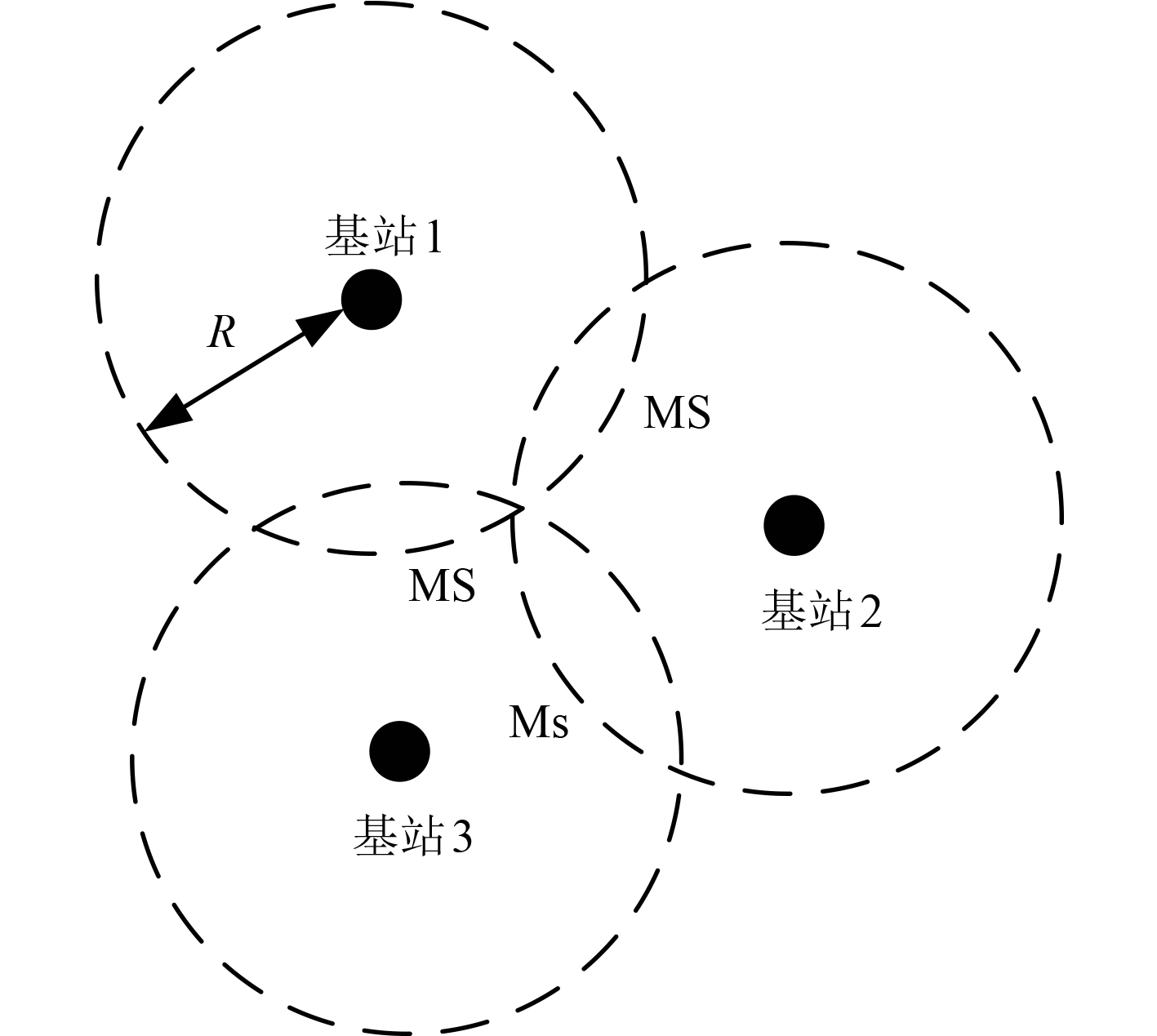图 2 TOA移动终端定位示意图 Fig. 2 Schematic diagram of TOA mobile terminal positioning

TOA定位技术中，终端与基站i之间的距离为：

 $R = c \times {t_i} \text{。}$

 $\left\{ {\begin{array}{*{20}{l}} {{R_1} = \sqrt {{{\left( {x - {x_1}} \right)}^2} + {{\left( {y - {y_1}} \right)}^2}} }，\\ {{R_2} = \sqrt {{{\left( {x - {x_2}} \right)}^2} + {{\left( {y - {y_2}} \right)}^2}} } \\ {{R_3} = \sqrt {{{\left( {x - {x_3}} \right)}^2} + {{\left( {y - {y_3}} \right)}^2}}} 。\end{array}} \right.$

2）TDOA定位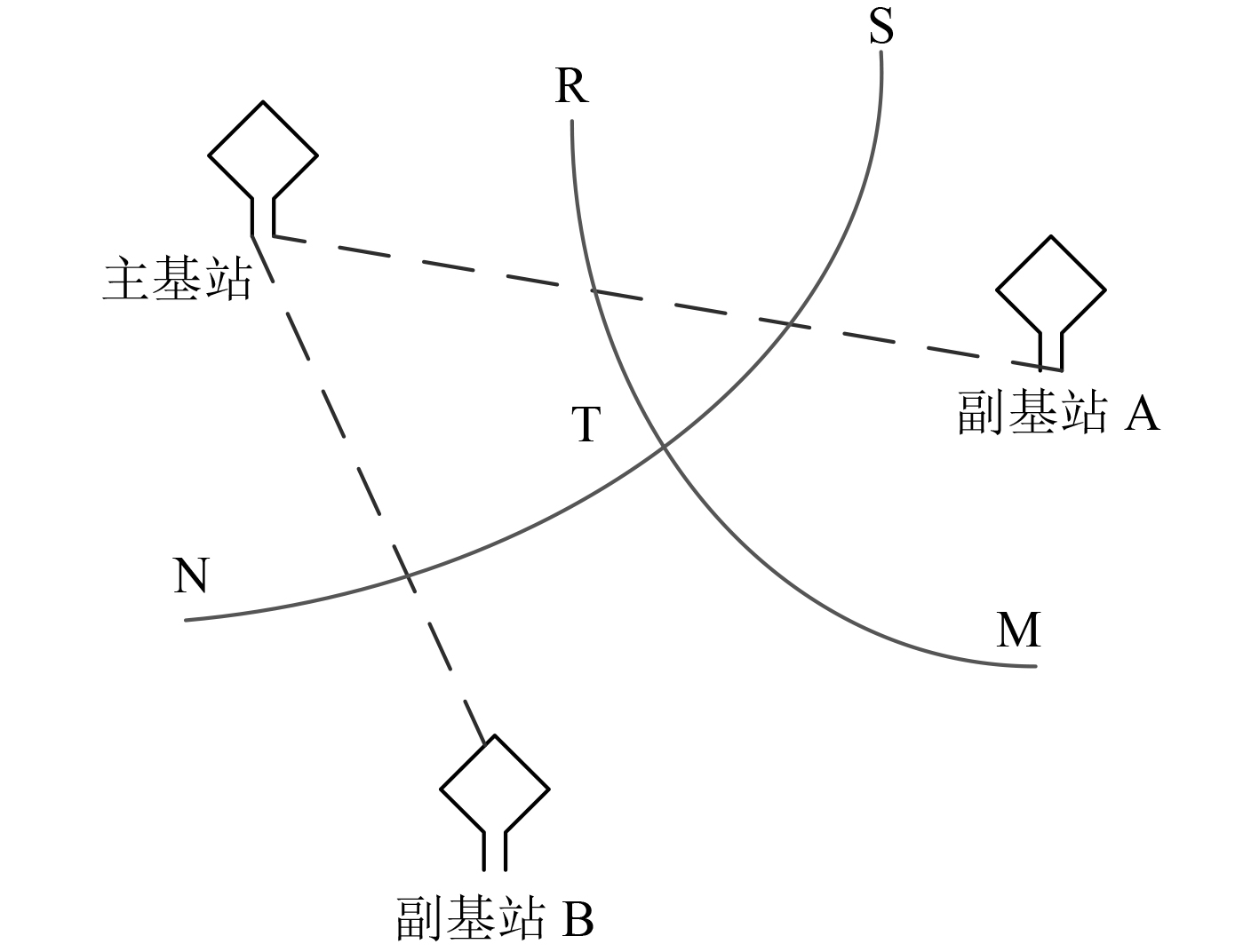图 3 TDOA定位技术示意图 Fig. 3 Schematic diagram of TDOA positioning technology

 $\left\{ {\begin{array}{*{20}{l}} {{R_{21}} = \sqrt {{{\left( {x - {x_2}} \right)}^2} + {{\left( {y - {y_2}} \right)}^2}} - \sqrt {{{\left( {x - {x_1}} \right)}^2} + \left( {y - {y_1}} \right)} } ，\\ {{R_{31}} = \sqrt {{{\left( {x - {x_3}} \right)}^2} + {{\left( {y - {y_3}} \right)}^2}} - \sqrt {{{\left( {x - {x_1}} \right)}^2} + \left( {y - {y_1}} \right)} } 。\end{array}} \right.$

1.3 基于CDMA通信链路的船舶终端定位技术

 $y(t) = \sum\limits_{i = - M}^M {\sum\limits_{k = 1}^K {\sum\limits_{l = 1}^L {{a_k}} } } {r_M}(t){b_k}[i]{S_k}\left( t \right){T_h} - {\tau _k} + \sigma n(t) \text{，}$

 ${S_k}(t) = \sum\limits_{i = 1}^N {{{( - 1)}^t}} {P_{{T_i}}}\left[ {t - (i - 1){T_\tau }} \right] \text{，}$

 $y(t) = \sum\limits_{i = - M}^M {\sum\limits_{k = 1}^K {\sum\limits_{l = 1}^L {{a_k}} } } {S_k}\left( t \right) + \sigma n(t) 。$
2 基于CDMA网络的船舶在线式监控系统开发 2.1 体系结构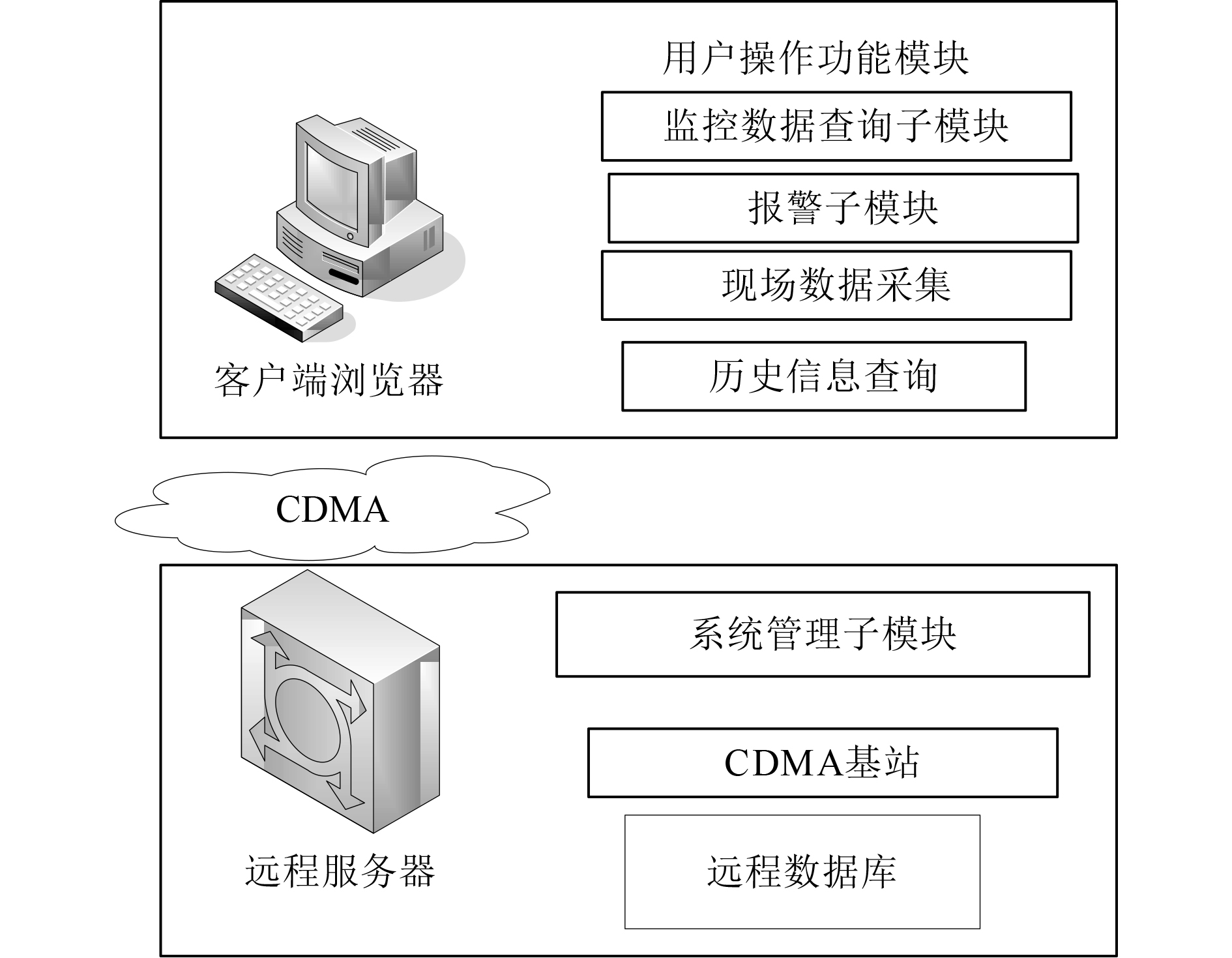图 4 舰船在线监控系统的体系结构设计 Fig. 4 Architecture design of ship online monitoring system

1）参数采集

2）参数降噪和处理

3）参数显示和预警

2.2 整体设计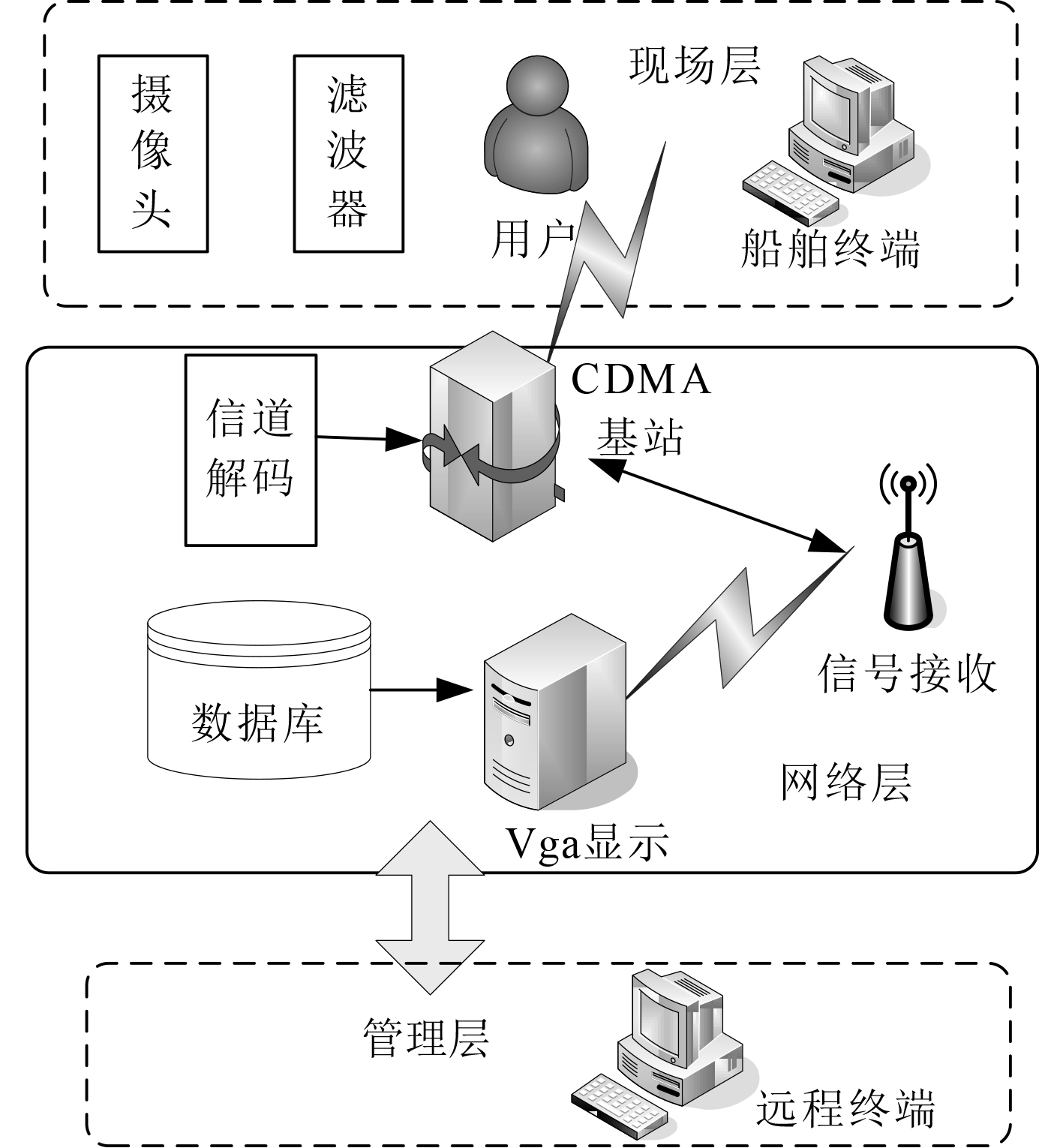图 5 舰船在线式监控系统原理图 Fig. 5 Schematic diagram of ship online monitoring system

1）网络层表 1 CDMA系统主要参数 Tab.1 Main parameters of CDMA system

2）管理层

3）现场层

2.3 关键部件设计

1）滤波器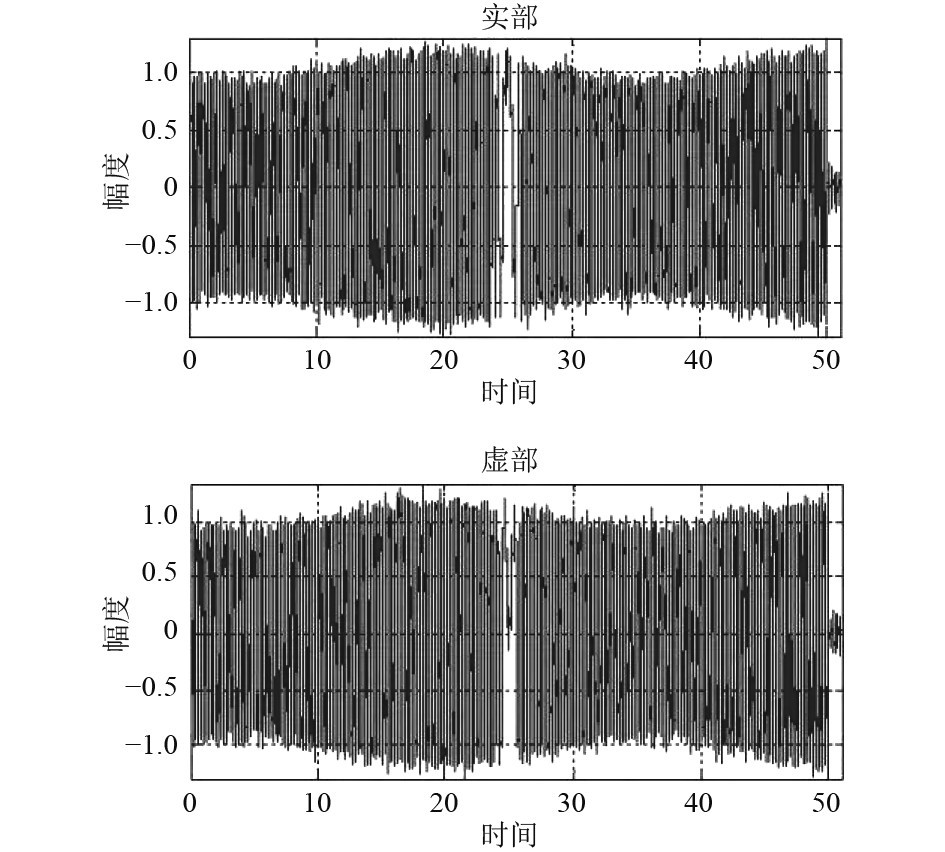图 6 高斯滤波器的滤波特性图 Fig. 6 Filtering characteristic diagram of Gaussian filter

 $F = 0.65\sqrt {\frac{{{C_{LPE}}}}{{{L_0}}}} \cdot {f_0} \cdot M \cdot {L_{LPE}} \text{，}$

 $\begin{gathered} M = \frac{{{f_0}}}{{{f_1}}}{\text{ = }}\frac{{50}}{{0.5\text{π} }}{\text{ = }}3056.3 \hfill，\\ {L_{LPE}} = \frac{{{L_0}}}{M}{\text{ = }}0.0045\;{\text{Hz}} \hfill \\ {C_{LPE}} = \frac{{{C_0}}}{M}{\text{ = }}0.166 。\end{gathered}$

2）LPC2378微处理器

3 结　语

  王翰康, 司马荣泽, 周显政, 等. 一种基于CDMA网络的海事数据传输系统[J]. 移动通信, 2005, 29(3): 91-94.  余桂芳, 余世明. 基于CDMA网络的售货机远程监控系统设计与实现[J]. 工业控制计算机, 2014 27(5): 33-35.  邱刚. 一种新型的准同步CDMA通信系统研究[J]. 武汉商业服务学院学报, 2002(z1): 179-181.  崔婧. 基于CDMA网络技术的水利水电监控系统的实现技术实际[J]. 城市建设理论研究(电子版), 2013(34).  邱玲, 周胜利, 梅刚, 等. W-CDMA无线多媒体通信系统设计考虑[J]. 通信学报, 1999, 20(10): 92.  黄百生, 卜淮原, 张瀚, 等. 一种北斗/CDMA导航系统数据融合的联邦卡尔曼滤波算法[J]. 后勤工程学院学报, 2009 25(1): 68−72  李刚健. 基于IP网络远程监控系统的设计与实现[J]. 微计算机信息, 2010, 26(4): 57-59.